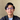# How to Search an Array in JavaScript

December 20, 2019

In the previous tutorial, we learn about how we can do CRUD operations to an array in JavaScript. In this tutorial, we are going to learn how to search a single item or multiple items inside an array.

Let's say that we have an array of todos.

``````const todos = [
"Learn React",
"Learn Java",
"Learn JavaScript",
"Learn Vue"
]``````

## Using find

The `find` method allows us to find a specific item inside an array based on the value. The callback should returns either true or false. You can read more about it here.

``todos.find((item) => item === "Learn JavaScript")``

Result:

``"Learn JavaScript"``

When we try to find an element that contains "Item" string, it will only return the first one that matches the condition.

``todos.find((item) => item.includes("Java"))``

Result:

``"Learn Java"``

## Using filter

The filter method most often used to search multiple items in a list. It returns a new array that only contains the items that match a specific condition. The callback should also returns a Boolean. Read more about it here.

``todos.filter((item) => item.includes("Java"))``

Result:

``````[
"Learn Java",
"Learn JavaScript"
]``````

If the condition only matches one item, it still returns a new array.

``todos.filter((item) => item === "Learn JavaScript")``

Result:

``["Learn JavaScript"]``

## Using forEach

Different than the `filter` method, the callback of `map` method expect nothing in return. This is very useful if you want to store your filtered value somewhere else in your code.

``````const filteredTodos = []

todos.forEach((item) => {
if (item.contains("Java")) {
filteredTodos.push(item)
}
})``````

Result:

``````[
"Learn Java",
"Learn JavaScript"
]``````